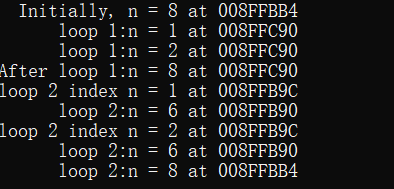# C语言存储类别和链接# C语言存储类别和链接​ 最近详细的复习C语言，看到存储类别的时候总感觉一些概念模糊不清，现在认真的梳理一下。C语言的优势之一能够让程序员恰到好处的控制程序，可以通过C语言的内存管理系统指定变量的作用域和生存周期，实现对程序的控制。

## 存储类别

• 基本概念

``````void fun(int n,double m);     同样可以声明为

void fun(int ,double );``````

## 存储期

C对象有4种存储期：

1) 静态存储期：如果一个对象具有静态存储期，那么它在程序的执行期间一直存在。文件作用域变量具有静态存储期，注意关键字`static`表明的是链接属性而不是存储期。以`static`声明的文件作用域变量具有内部链接，无论具有内部链接还是外部链接，所有的文件作用域变量都具有静态存储期。

``````int fun(int num)
{
static int Index;
...
}``````

2) 线程存储期：用于并发程序设计，一个程序的执行可以分为多个线程，具有线程存储期的变量从被声明时到线程结束一直存在。以关键字`_Thread_local`声明一个对象时，每个线程都获得该变量的私有备份。

3) 自动存储期：块作用域变量通常具有自动存储期，当程序进入定义这些变量的块时，会为这些变量分配内存，当程序离开这个块时会自动释放变量占用的内存，这种做法相当于把自动变量占用的内存视为可重复利用的工作区或暂存区。

4) 动态分配存储期

## 五种存储类别

• 五种存储类别

• 自动变量

``````void hiding()
{
int x = 30;
printf("x in outer block: %d at %p\n", x, &x);
{
x = 77;
printf("x in inner block: %d at %p\n", x, &x);
}
// 块中内存被释放隐藏的x恢复 x = 30
printf("x in outer block: %d at %p\n", x, &x);

while (x++ < 33)
{
int x = 100;
x++;
printf("x in while loop: %d at %p\n", x, &x);
}
printf("x in outer block: %d at %p\n", x, &x);
}
``````

``````void forc()
{
int n = 8;
printf("  Initially, n = %d at %p\n", n, &n);
for (int n = 1; n < 3; ++n)
printf("      loop 1:n = %d at %p\n", n &n);
// 离开循环后原始的你又起作用了
printf("After loop 1:n = %d at %p\n", n &n);
for (int n = 1; n < 3; ++n)
{
printf("loop 2 index n = %d at %p\n", n, &n);
// 重新初始化的自动变量，作用域没有到循环里的n
int n = 6;
printf("      loop 2:n = %d at %p\n", n, &n);
// 起作用的仍然是循环中的n
n++;
}
// 离开循环后原始的n又起作用了
printf("      loop 2:n = %d at %p\n", n, &n);
}``````• 寄存器变量

• 块作用域的静态变量

​ 首先要明确概念静态变量并不是指值不改变的变量，而是指它在内存中的位置不变。具有文件作用域的静态变量自动具有静态存储期。

``````void trystat();

int main()
{
int count = 1;
for (count = 1; count <= 3; count++)
{
printf("Here comes iteration %d:\n", count);
trystat();
}
trystat();
return 0;
}

void trystat()
{
static int stay = 1;
}``````

​ 可以看出每次离开块fade变量的值都会被重新的初始化，而stay只是在编译函数`void trystat()`的时候被初始化了一次,在离开自己函数体的块和for循环块之后都会递增，说明stay访问的对象一直存在并没有像自动变量一样被释放掉。

• 外部链接的静态变量

​ 具有外部链接、静态存储期和文件作用域，属于该类别的变量属于外部变量。只需要把变量的声明放在所有函数的外面就创建了一个外部变量。为了表明该函数使用了外部变量，需要使用关键字`extern`来再次申明。如果在一个源文件中使用的外部变量声明在了另一个源文件中，则必须要使用extern来申明。

• 内部链接的静态变量

``````static int val = 1;
int main()
{
...
}``````

``````int global = 1;
static int local_global = 2;
int main
{
extern int global = 1;
extern int local_global = 2;
...
}``````• 回顶